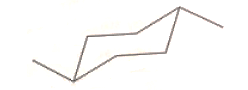# Problem: The molecular formula for the condensed structural formula of the hydrocarbon below is: a. C6H10 b. C8H15 c. C8H30 d. C5H30 e. C8H16

###### FREE Expert Solution
92% (456 ratings)
###### Problem Details

The molecular formula for the condensed structural formula of the hydrocarbon below is:

a. C6H10

b. C8H15

c. C8H30

d. C5H30

e. C8H16Frequently Asked Questions

What scientific concept do you need to know in order to solve this problem?

Our tutors have indicated that to solve this problem you will need to apply the Empirical Formula concept. You can view video lessons to learn Empirical Formula. Or if you need more Empirical Formula practice, you can also practice Empirical Formula practice problems.

What is the difficulty of this problem?

Our tutors rated the difficulty ofThe molecular formula for the condensed structural formula o...as medium difficulty.

How long does this problem take to solve?

Our expert Chemistry tutor, Dasha took 3 minutes and 54 seconds to solve this problem. You can follow their steps in the video explanation above.